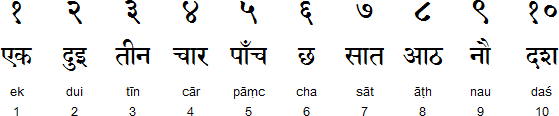# Convert English Number To Nepali Numbers Via Wp FunctionsPublished On Thursday June 4, 2020

There is a simple technique to convert English numbers to Nepali numbers in WordPress via a custom function.

The Custom function to be inserted in function PHP file is:

```//for converting eng number into nepali number
function EnToNp(\$string) {
\$array = array("0" => "०","1" => "१","2" => "२","3" => "३","4" => "४","5" => "५","6" => "६","7" => "७","8" => "८","9" => "९");
return strtr(\$string,\$array);
}```

Now to use the function we can simply use the function as:

`<?php echo EnToNp("9");?>`

As per the above example, as a result of the method EnToNp, the number is converted to Nepali format. This is how we can simply achieve the result.

0 0 vote
Article Rating
Subscribe
Notify of